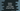# Python program to iterate over a tuple using a for loop## Python program to iterate over a tuple using a for loop:

for loop can be used to iterate over a tuple. A tuple returns an iterator in python and we can iterate through its content by using a for loop. In this post, I will show you how to iterate over a tuple using a for loop and different examples on this.

## Example 1: Iterate through the items of a tuple:

Let’s take one small example that shows how to iterate through the items of a tuple that includes string values.

``````given_tuple = ('one', 'two', 'three', 'four', 'five')

for item in given_tuple:
print(item)``````

If you run it, it will print the below output:

``````one
two
three
four
five``````

As you can see here, we can iterate through the items of the tuple using the for-in loop.

## Example 2: Iterating the items of a tuple of numbers:

In a similar way, we can also iterate through a number tuple, i.e. a tuple holding only numbers:

``````given_tuple = (1, 2, 3, 4, 5, 6, 7, 8, 9, 10)

for item in given_tuple:
print(item)``````

It will print:

``````1
2
3
4
5
6
7
8
9
10``````

## Example 3: Iterating the items of a tuple with different types of values:

We can iterate through a tuple that holds different types of elements like string, number etc. Let’s take a look at the below program:

``````given_tuple = (1, 'Two', 3, 'Four', 5, 'Six', 7, 8, 'Nine', 10)

for item in given_tuple:
print(item)``````

It will print:

``````1
Two
3
Four
5
Six
7
8
Nine
10``````

As you can see, we can iterate through the items of a tuple using a for loop irrespective of the content type.